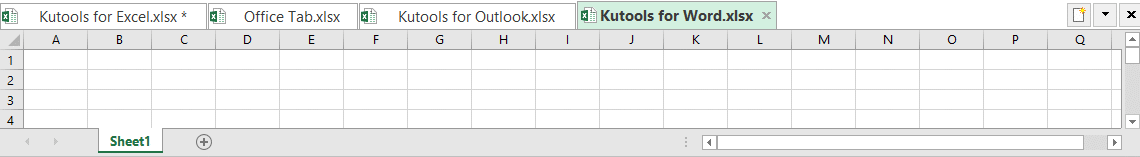## Calculate the difference between two dates in days, weeks, months and years in Excel

When dealing with the dates in a worksheet, you may need to calculate the difference between two given dates for getting the number of days, weeks, months or years. This article, I will talk about how to solve this task in Excel.

#### Calculate the difference between two given dates in days

To get the number of days between two given dates, please use below formulas:

1. Enter any one of the below formulas into a blank cell where you want to get the result:

=DATEDIF(A2,B2,"D")
=B2-A2

Note: In the above formulas: A2 is the start date cell and B2 is the end date cell.

2. Then, drag the fill handle down to the cells to fill this formula, and the number of days between the two dates has been calculated as below screenshot shown: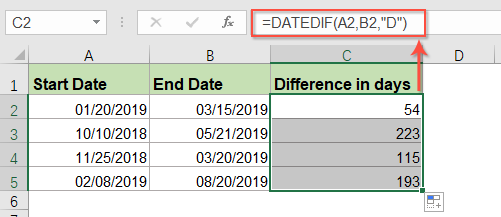#### Calculate the difference between two given dates in months

If you want to get the number of months between two specific dates, please enter the following formula into a blank cell:

=DATEDIF(A2,B2,"M")

Note: A2 is the start date cell and B2 is the end date cell.

And then, drag the fill handle down to the cells to apply this formula, and the difference between two dates in months has been calculated, see screenshot: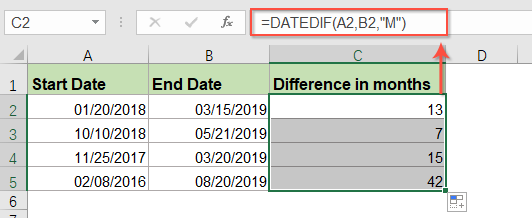#### Calculate the difference between two given dates in years

To calculate the number of years between two dates, the DATEDIF function also can help you.

Please enter the below formula into a blank cell:

=DATEDIF(A2,B2,"Y")

Note: A2 is the start date cell and B2 is the end date cell.

And then, drag the fill handle down to the cells to apply this formula, and the number of years between the specific two dates has been returned, see screenshot: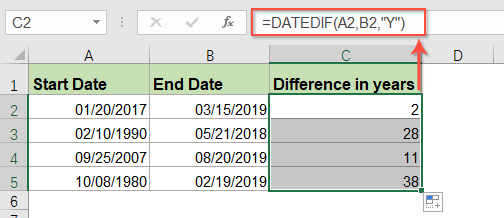#### Calculate the difference between two given dates in days, months and years

If you need to calculate the difference between two dates in days, months and years together, you should apply the below formula:

=DATEDIF(A2, B2, "y") &" years, "&DATEDIF(A2, B2, "ym") &" months, " &DATEDIF(A2, B2, "md") &" days"

Note: A2 is the start date cell and B2 is the end date cell.

And then drag the fill handle down to the cells that you want to apply this formula, then, you will get the following result: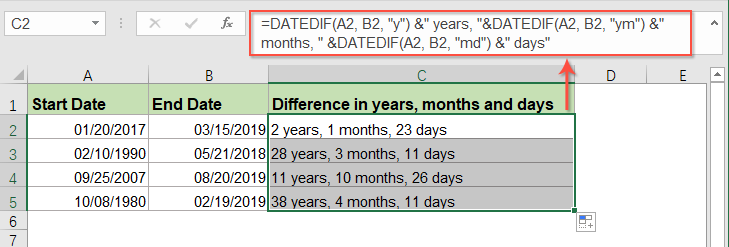#### Calculate the difference between two given dates in weeks

The DATEDIF function also can help you to calculate the number of weeks between two dates, please use the following formula into a blank cell:

=(DATEDIF(A2,B2,"D")/7)

Note: A2 is the start date cell and B2 is the end date cell.

Then, drag the fill handle down to the cells that you want to apply this formula, and you will get the weeks in decimal numbers between two dates, see screenshot: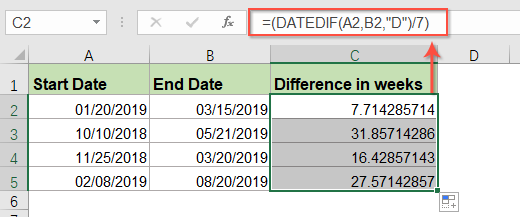• TipsIf you want to get the number of full weeks between two dates, please apply this formula:
• =ROUNDDOWN((DATEDIF(A2, B2, "d") / 7), 0)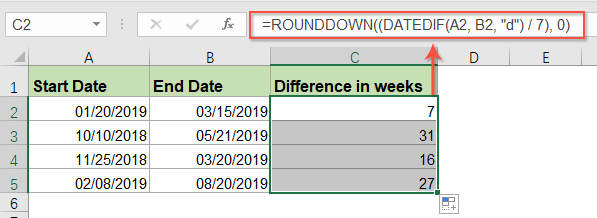#### Calculate the difference between two given date times in days, hours and minutes

If you have two lists of date and times, to calculate the difference between two date times in days, hours and minutes, the following formula can do you a favor.

Please copy and paste the below formula into a blank cell:

=INT(B2-A2)&" days "&TEXT(B2-A2,"h"" hours ""m"" minutes """)

Note: A2 is the start date time cell and B2 is the end date time cell.

And then, drag the fill handle down to the cells that you want to apply this formula, and you will get the following result as you need: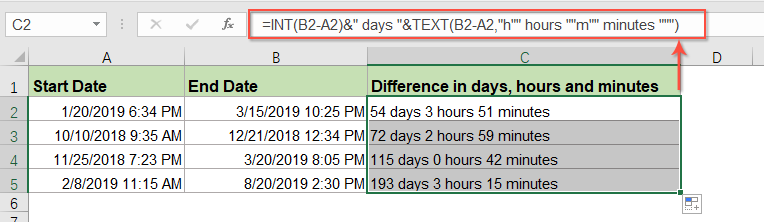#### More relative date and time articles:

• Calculate Hours Between Times After Midnight In Excel
• Supposing you have a time table to record your work time, the time in Column A is the start time of today and time in Column B is the end time of the following day. Normally, if you calculate the time difference between the two times by directly minus "=B2-A2", it will not display the correct result as left screenshot shown. How could you calculate the hours between two times after midnight in Excel correctly?
• Calculate Working Hours Per Month In Excel
• Normally, most of us may work 8 hours a day, 5 days a week. Here, I want to get the total working hours per month for calculating the salary. How to calculate the total working hours per month in Excel?
• Calculate Hours Worked And Minus Lunch Time In Excel
• As a company employee, we need punch in and punch out every work day, counting the total worked time and minus the lunch time of a day can help to calculate salary according to the time. For example, I have the following daily attendance record which contains log in, log out and lunch start, lunch end times in a worksheet, now, I want to calculate the total hours worked but minus lunch time each day. To finish this job, I can introduce some formulas for you.

## The Best Office Productivity Tools

### Kutools for Excel Solves Most of Your Problems, and Increases Your Productivity by 80%

• Super Formula Bar (easily edit multiple lines of text and formula); Reading Layout (easily read and edit large numbers of cells); Paste to Filtered Range...
• Merge Cells/Rows/Columns and Keeping Data; Split Cells Content; Combine Duplicate Rows and Sum/Average... Prevent Duplicate Cells; Compare Ranges...
• Select Duplicate or Unique Rows; Select Blank Rows (all cells are empty); Super Find and Fuzzy Find in Many Workbooks; Random Select...
• Exact Copy Multiple Cells without changing formula reference; Auto Create References to Multiple Sheets; Insert Bullets, Check Boxes and more...
• Favorite and Quickly Insert Formulas, Ranges, Charts and Pictures; Encrypt Cells with password; Create Mailing List and send emails...
• Extract Text, Add Text, Remove by Position, Remove Space; Create and Print Paging Subtotals; Convert Between Cells Content and Comments...
• Super Filter (save and apply filter schemes to other sheets); Advanced Sort by month/week/day, frequency and more; Special Filter by bold, italic...
• Combine Workbooks and WorkSheets; Merge Tables based on key columns; Split Data into Multiple Sheets; Batch Convert xls, xlsx and PDF...
• Pivot Table Grouping by week number, day of week and more... Show Unlocked, Locked Cells by different colors; Highlight Cells That Have Formula/Name...### Office Tab - brings tabbed interface to Office, and make your work much easier

• Enable tabbed editing and reading in Word, Excel, PowerPoint, Publisher, Access, Visio and Project.
• Open and create multiple documents in new tabs of the same window, rather than in new windows.
• Increases your productivity by 50%, and reduces hundreds of mouse clicks for you every day!